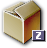# The site's hierarchy

Mar 2009
MTWTFSS
2324252627281
2345678
9101112131415
16171819202122
23242526272829
303112345
News
• Les premiers zoom de galaxies sont accessibles
• Le halo FOF 6133 de la boite Horizon L et le halo FOF 544 de Horizon S ont été resimulés avec plusieurs techniques de zoom. Il est important que chaque "zoomer" valide sa méthode, avant de lancer une campagne de zoom sur un plus grand cataloque.
• Les données de la simulation Mare Nostrum sont disponibles
• 34 snapshots jusqu’à z=4 sont accessibles sur horizon3 et sur le serveur de fichiers de l’IDRIS à la collaboration Horizon.
• Méso Machine HPC1 opérationnelle
• Depuis le 23 Octobre 2005, la Méso machine du site horizon est operationnelle. Elle correspond à 3 quadriprocesseurs avec chacun 64 Giga de RAM reliés par infiniband, ainsi qu’un access conséquent (sur une base de projet dédié) au reste de la ferme). Son acces est ouvert a toute personne de la collaboration ayant acces à la minigrille et qui en fait la demande a admin-minigrille
• http://
• Workshop Horizon le 14 et 15 novembre 2005
• Il aura lieu à Paris les 14 et 15 novembre 2005 (prévoir une nuit sur place). L’enregistrement est ouvert dans la rubrique "meeting!"
• http://

# From Jeans Equations

by Emsellem Eric (Monday 3 April 2006)

One other way to generate velocities is to use the velocity moments (as compared to the full distribution functions). For a rather large range of models (spherical, axisymmetric, triaxial, multi-components), it is possible to derive the first two velocity moments along each principal axis (e.g. R, Z for cylindrical coordinates). We can then simply assume any simple functional form which shares the same moments to generate velocities consistently with the assumed dynamical model.

A common practice in this context is to assume a Gaussian distribution with its mean being the first velocity moment (the mean velocity in this direction) and its root mean square being its second (centred) velocity moment, namely the stellar velocity dispersion along that direction.

For each particle, it is then easy to generate the corresponding velocities:first get the position of the particle (e.g. R, z, theta)then draw randoms numbers using the mean velocities and dispersions along these directions at that specific position. One thing to remember is to reject velocities which are above the local escape velocity (\$\sqrt2 \phi).

To illustrate this method, we attach here a python routine which includes modules to make an N body realization of a so-called MGE (Multi-Gaussian Expansion) model. This model is just a representation for a luminosity or mass model using a sum of tri-dimensuonal Gaussians; This simplifies the derivation of the gravitational potential, and allows the analytical derivation of the projected luminosity for any viewing angle. See Monnet et al. 1992, A&A 253, 366 and Emsellem et al. 1994, A&A 285, 723 for details (one application of this formalism to the dynamical modeling of galaxies can be found in Emsellem et al. 1999, MNRAS 303, 495).

So the steps to generate a set of particles with their positions and velocities is rather simple. First set up the parameters for an MGE model of your choice. This is done using the MGE class and specifying a specific ascii file where the parameters are provided (an example of such an ascii file is provided below). Then you just generate the positions/velocities using the function init_nbody.

This would look like (# are comments): # use the mge module

%run mge.py

# reads the ascii file and initialise the model

model = MGE("MGE_N3377.mge")

# make the N body realization

This will provide 100 000 particles with a cut-off in Z and R of 10 kpc and a Satoh parameter (setting the anisotropy of your model) of 1 (meaning here = isotropy). Details can be found in the papers mentioned above, or in the python routine (and otherwise, use emails...).

An example of an MGE ascii file can be found here:MGE_N3377.mge
Ascii file including the MGE parameters for the model of a galaxy (here: NGC 3377).

and the python module can be found there:mge.py
This is a python module which allows you to make an N body realization consistent with a Multi-Gaussian Expansion model.